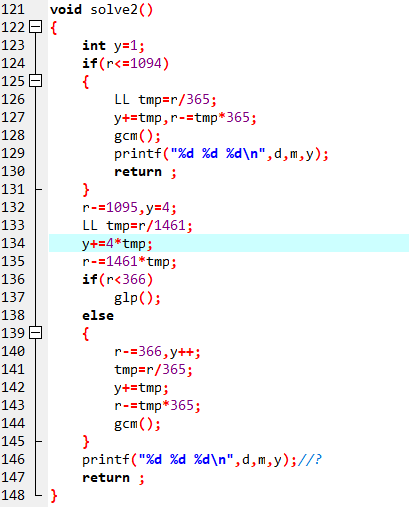# CSP2020游记--跻攀分寸不可上,失势一落千丈强

#### 引言

$CSP$之后马上是紧锣密鼓的期中考试，所以消失了一周没有去竞赛那边。

#### Day 1

$T1$开题，极度不舒适。$T3$看起来是个数据结构题，但是我没有什么思路（不会算答案），所以先去做了$T4$

$T4$用了两个优先队列进行维护，总体思想就是如果这条蛇吃了别人之后自己会被吃掉，就不吃。

$T2$先打了个暴力，然后还是没有什么比较好的思路，时间不是很多了，又心心念念着$T1$，然后就去肝$T1$

$By\ the\ way,$其实说是在准备半期，周日的时候还抽了几个小时时间出来赶稿子（但不得不说$ddl$真的是第一生产力），明明$5$个月前就开始构思了的，但偏偏实在截稿日期前$2$周才开始动笔，并且最后$4$天完成了$80\%$，拖延症真的是无药可救。第一次尝试写科幻，但是我觉得自己写得有亿点崩，叙事也没有安排好，而且还有点...有点中二？希望审稿人没事。由于害怕入不了围，所以还写了一个自己比较擅长的风格的散文。（果然擅长的就是不一样，$1.5h$能肝将近$3k$字（主要是还加上了$ddl$$buff$，这个平时想想都不敢想））

#### Day 8

$T2$莫名奇妙挂了一个点，然后发现位数如果全都可行的话就是$2^{64}-n$,而$2^{64}$已经炸$ULL$了，所以这种情况要特判。（这是我妹有想到的，希望$CCF$的数据善良

$T3$稳拿暴力分，$T4$果然只有$n==3$的部分分啊。

#### Day 10

posted @ 2020-11-15 15:09  Starlight_Glimmer  阅读(45)  评论(0编辑  收藏